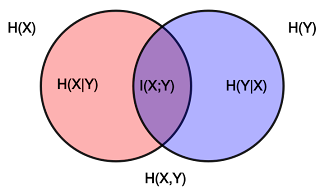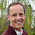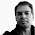## Saturday, June 29, 2013

### What is Information? (Part 4: Information!)

This is the 4th (and last) installment of the "What is Information" series. The one where I finally get to define for you what information is. The first three parts can be found here: Part 1, Part 2, Part 3.

Let me quickly summarize the take-home points of parts1-3, just in case you are reading this temporally removed from parts1-3.

1.) Entropy, also known as "uncertainty", is something that is mathematically defined for a "random variable". But physical objects aren't mathematical. They are messy complicated things. They become mathematical when observed through the looking glass of a measurement device that has a finite resolution. We then understand that a physical object does not "have an entropy". Rather, its entropy is defined by the measurement device I choose to examine it with.  Information theory is a theory of the relative state of measurement devices.

2.) Entropy, also known as uncertainty, quantifies how much you don't know about something (a random variable). But in order to quantify how much you don't know, you have to know something about the thing you don't know. These are the hidden assumptions in probability theory and information theory. These are the things you didn't know you knew.

3.) Shannon's entropy is written as "p log p", but these "p" are really conditional probabilities if you know that they are not uniform (all p the same for all states). They are not uniform given what else you know. Like that they are not uniformly distributed. Duh.

Alright, now we're up to speed. So we have the unconditional entropy, which is the one where we know nothing about the system that the random variable describes. We call that $H_{\rm max}$, because an unconditional entropy must be maximal: it tells us how much we don't know if we don't know anything. Then there is the conditional entropy $H=-\sum_i p_i\log p_i$, where the $p_i$ are conditional probabilities. They are conditional on some knowledge. Thus, $H$ tells you what remains to be known. Information is "what you don't know minus what remains to be known given what you know". There it is. Clear?

"Hold on, hold on. Hold on for just a minute"

What?

"This is not what I've been reading in textbooks."

"Don't condescend. What I mean is that this is not what I would read in, say, Wikipedia, that you link to all the time. For example, in this link to `mutual information'. You're obviously wrong.
Because, you know, it's Wiki!"

So tell me what it is that you read.

"It says there that the mutual information is the difference between the entropy of random variable $X$,  $H(X)$, and the conditional entropy $H(X|Y)$, which is the conditional entropy of variable $X$ given you know the state of variable $Y$."

"Come to think of it, you yourself defined that conditional entropy at the end of your part 3. I think it is Equation (5) there!" And there is this Venn diagram on Wiki. It looks like this:"Source: Wikimedia
Ah, yes. That's a good diagram. Two variables $X$ and $Y$. The red circle represents the entropy of $X$, the blue circle the entropy of $Y$. The purple thing in the middle is the shared entropy $I(X:Y)$, which is what $X$ knows about $Y$. Also what $Y$ knows about $X$. They are the same thing.

"You wrote $I(X:Y)$ but Wiki says $I(X;Y)$. Is your semicolon key broken?"

Actually, there are two notations for the shared entropy (a.k.a information) in the literature. One uses the colon, the other the semicolon. Thanks for bringing this up. It confuses people. In fact, I wanted to bring up this other thing....

"Hold on again. You also keep on saying "shared entropy" when Wiki says "shared information". You really ought to pay more attention."

Well, you. That's a bit of a pet-peeve of mine. Just look at the diagram above. The thing in the middle, the purple area, it's a shared entropy. Information is shared entropy. "Shared information" would be, like, shared shared entropy. I think that's a bit ridiculous, don't you think?

"Well, if you put it like this. I see your point. But why do I read "shared information" everywhere?"

That is, dear reader, because people are confused about what to call entropy, and what to call information. A sizable fraction of the literature calls what we have been calling "entropy" (or uncertainty) "information". You can see this even in the book by Shannon and Weaver (which, come to think of it, was edited by Weaver, not Shannon). When you do this, then what is shared by the "informations" is "shared information". But that does not make any sense, right?

"I don't understand. Why would anybody call entropy information? Entropy is what you don't know, information is what you know. How could you possibly confuse the two?"

I'm with you there. Entropy is "potential information". It quantifies "what you could possibly know". But it is not what you actually know. I think, between you and me, that it was just sloppy writing at first, which then ballooned into a massive misunderstanding. Both entropy and information are measured in bits, and so people would just flippantly say: "a coin has two bits of information", when they mean to say "two bits of entropy". And it's all downhill from there.

I think I've made my point here, I hope. Being precise about entropy and information really matters. Colon vs. semicolon does not. Information is "unconditional entropy minus conditional entropy". When cast as a relationship between two random variables $X$ and $Y$, we can write it as

$I(X:Y)=H(X)-H(X|Y)$.

And because information is symmetric in the one who measures and the one who is being measured (remember: a theory of the relative state of measurement devices...) this can also be written as

$I(X:Y)=H(Y)-H(Y|X)$.

And both formulas can be verified by looking at the Venn diagram above.

"OK, this is cool."

"Hold on, hold on!"

What is it again?

"I just remembered. This was all a discussion that came after I brought up Wikipedia, that says that information was $I(X:Y)=H(X)-H(X|Y)$, while you said it was $H_{\rm max}-H$, where the $H$ was clearly an entropy that you write as $H=-\sum_i p_i\log p_i$. All you have to do is scroll up, I'm not dreaming this!"

So you are saying that textbooks say

$I=H(X)-H(X|Y)$  (1)

while I write instead

$I=H_{\rm max}-H(X)$,   (2)

where $H(X)=-\sum_i p_i\log p_i$. Is that what you're objecting to?

"Yes. Yes it is."

Well, here it is in a nutshell. In (1), information is defined as the difference between the actual observed entropy of $X$, minus the actual observed entropy of $X$ given that I know the state of $Y$ (whatever that state may be).

In (2), information is defined as what I don't know about $X$ (without knowing any of the things that we may implicitly know already), and the actual uncertainty of $X$. The latter does not mention a system $Y$. It quantifies my knowledge of $X$ without stressing what it is I know about $X$. If the probability distribution with which I describe $X$ is not uniform, then I know something about $X$. My $I$ in Eq. (2) quantifies that. Eq. (1) quantifies what I know about $X$ above and beyond what I already know via Eq. (2). It quantifies specifically the information that $Y$ conveys about $X$. So you could say that the total information that I have about $X$, given that I also know the state of $Y$, would be

$I_{\rm total}=H_{\rm max}-H(X) + H(X)-H(X|Y)=H_{\rm max}-H(X|Y)$.

So the difference between what I would write and what textbooks write is really only in the unconditional term: it should be maximal. But in the end, Eqs. (1) and (2) simply refer to different informations. (2) is information, but I may not be aware how I got into possession of that information. (1) tells me exactly the source of my information: the variable $Y$.

Isn't that clear?

"I'll have to get back to you on that. I'm still reading. I think I have to read it again. It sort of takes some getting used to."

I know what you mean. It took me a while to get to that place. But, as I has hinted at in the introduction to this series, it pays off big time to have your perspective adjusted, so that you know what you are talking about when you say "information". I will be writing a good number of blog posts that reference "information", and many of those are a consequence of research that was only possible when you understand the concept precisely. I wrote a series on information in black holes already (starting here). That's just the beginning. There will be more to come, for example on information and cooperation. I mean, how you can only fruitfully engage in the latter if you have the former.

I know it sounds more like a threat than a promise. I really mean it to be a promise.

1.Your concept of entropy is natural to a probability theorist, but not so much to most physicist, I would guess. Most physicists appear to assign real physical significance to the concept of entropy. When a physicist thinks about the second law of thermodynamics, which states that entropy in a closed system increases with time, she does not just think that this is a restatement of the fact that we loose information about the state of the system as time goes on, but rather she thinks that this says something about the actual physical system itself. For example physicists talk about the "heat death" of the universe; the idea that when the universe reaches maximum entropy that then no physical processes can take place any more. Am I right that you would say that this idea is misguided? That the fact that we have lost all information about a system does not mean that the system itself has changed its behaviour in any way? That the fact that we, due to our lack of information, can no longer extract work from the system does not imply that the system itself dies?

1.The way you describe how most physicists think about the 2nd law is, I think, precisely right. They DO think that something happens, when in fact they just lose information. You can push this to the extreme by the way, if you believe that the universe has a wavefunction. Then you replace the 2nd law by the statement that the entropy of the universe is constant (and zero) for all time, but that the local (apparent) entropy increases, mostly due to the fact that the universe expands. The 2nd law is one of the silliest "laws" of all of physics. It does not exist. It is a consequence of not understanding information theory. Can't blame Boltzmann, though. But Feynman should have figured it out. He knew Shannon, but I don't think he read his work.

2.Chris, it is nice to hear someone say that so bluntly.

3.Is this to say that entropy in the context of information is a different beast from entropy in the context of thermodynamics? It seems they are extremely analogous but strain to meet at the ends.

Also I'm curious if you could expand on this:

"The 2nd law is one of the silliest "laws" of all of physics. It does not exist. It is a consequence of not understanding information theory."

See I have my thermodynamics exam coming up, and this sort of shakes my ground a bit.

Thank you

4.Chris, I would also be interested in hearing more about what you mean when saying that the second law does not exist. It is a fact, is it not, that it depends on the particular dynamics that govern a system whether entropy increases or decreases. For example in a system that is described by an absorbing Markov chain with an absorbing state, entropy decreases with time as the probability becomes more and more concentrated in that state. The second law of thermodynamics states that nature is not described by such a system but by one in which entropy increases. In what sense does that law not exist?

5.OK, I see that I may have to devote an entire blog post to the relationship between thermodynamic and Shannon entropy, as I get asked this a lot. So, in anticipation of that post, here's the short version. The quantitiy that is increasing when non-equilibrium systems "equilibrate themselves" is a conditional entropy. The unconditional entropy must stay constant. That is why the 2nd law does not exist. And the fact that the conditional entropy increases (in just the way that Boltzmann observed) is just a triviality. Whereas in standard thermodynamics, it was a mystery. Well, it was a mystery because it was wrong.

6.Chris, why do you say that it is a triviality that the conditional entropy increases. As the example of the absorbing Markov chain seems to show, in some systems the conditional entropy decreases with time. The conditional entropy I am thinking of here is H(X(t)|X(0)) where X(t) describes the state of the chain at time t. If it is an absorbing chain with a single absorbing state, the probability distribution for X(t) becomes more and more concentrated on that one state as time progresses and hence the entropy decreases with time.

7.Furthermore I find it confusing that you say that the unconditional entropy must stay constant. The unconditional entropy H(X(t)) of some stochastic process X(t) does not usually stay constant. It appears that you use the term "conditional entropy" in a different way from others in that you call the entropy H(X) of any random variable X "conditional" unless X is uniformly distributed. Is that correct? Do you not find that confusing?

8.Hi Gustav,

the entropy of a Markov process (certainly a well-defined thing that I also have written about) is not the same thing as the thermodynamical entropy of a system. In fact, the 2nd law has no chance of governing the entropy of a Markov chain, because that depends (as you correctly point out) on the dynamics of this process, and if you have an absorbing boundary condition that entropy could even vanish. A thermodynamical system is different. In a sense, the transition rules for the Markov process describing the physical system variable is very special. The reason why the 2nd law is trivial when written in terms of a conditional entropy is that if you don't measure anything, then all that can happen to you as a system equilibrates is that you lose information. And that makes the conditional entropy go up. As I said, I should write an entire post about all that. I wrote about it a little bit in http://arxiv.org/abs/1112.1941

9.The reason I gave the example of the absorbing Markov chain is to illustrate that the statement that "if you don't measure anything, then all that can happen to you as a system equilibrates is that you lose information" is not trivial. It holds for the kinds of processes one studies in thermodynamics (ergodic, detailed balance) but not in general Markov processes and it is not trivial to explain why, or is it?

10.The trivial explanation would be Liouville's theorem in classical mechanics, or unitarity in quantum mechanics. In short, the process must be reversible. The fact that no two microstates evolve to the same microstate means that the system cannot do any concentration for you, you have to do it yourself.

2.I think you flipped eq (1) and (2) in the sentence: "(1) is information, but I may not be aware how I got into possession of that information. (2) tells me exactly the source of my information: the variable Y."

As for the distinction... I sort-of disagree. I don't think (2) is really all that necessary, though of course I could be wrong. What I assert is that:
*The information content of a system is not defined except with respect to some other system.*

Essentially, I'm happy just avoiding the need for a definition of non-conditional information. Yeah, it is logically there... It is just the information of X conditional with everything else you know about in the universe, but that doesn't seem particularly useful to me.

3.PS: I move (in the parliamentary sense) that we just abolish the use of the term "entropy" outside the realm of thermodynamics (as in S). "Uncertainty" seem to cause a lot less confusion.

4.I second that motion.